# R S Aggarwal Solutions for Class 10 Maths Chapter 4 Quadratic Equations Exercise 4D

R S Aggarwal Solutions for Class 10 Maths exercise 4d contains detailed step-by-step explanations to all the problems enlisted under the exercise. This exercise concept helps students to find the nature of roots of a quadratic equation. Students can download free pdf of class 10 Maths Chapter 4 quadratic equations exercise 4d by clicking on the given link below.

## Download PDF of R S Aggarwal Solutions for Class 10 Maths Chapter 4 Quadratic Equations Exercise 4D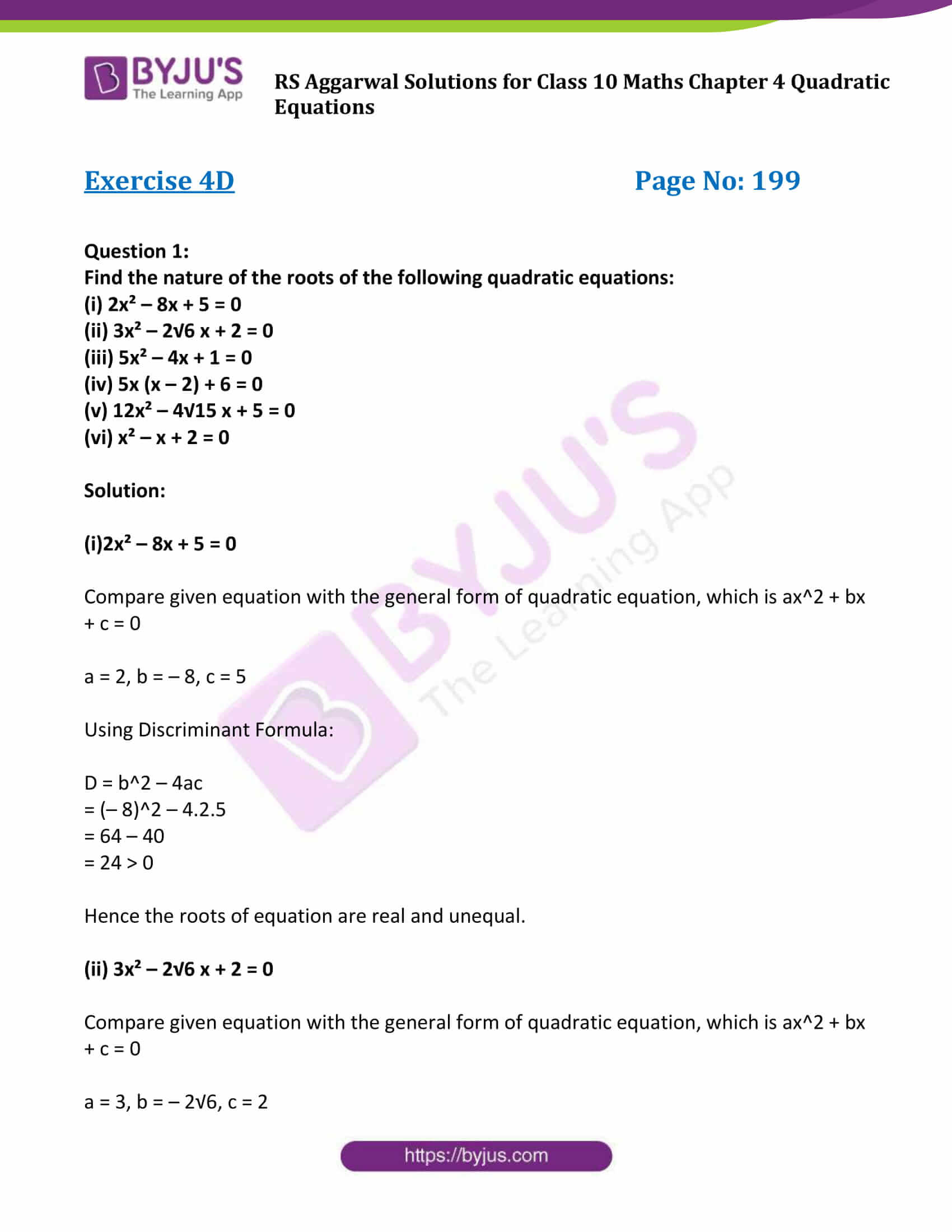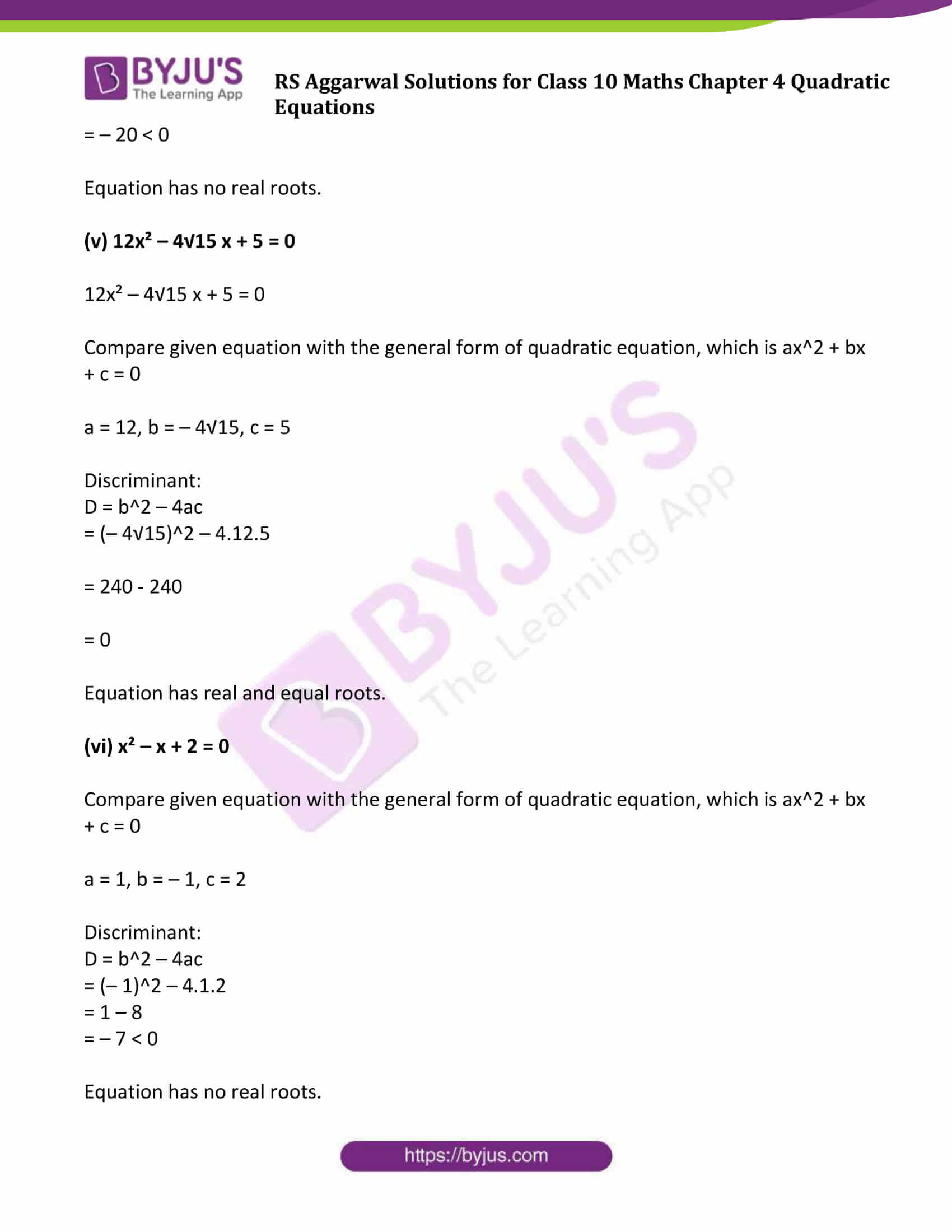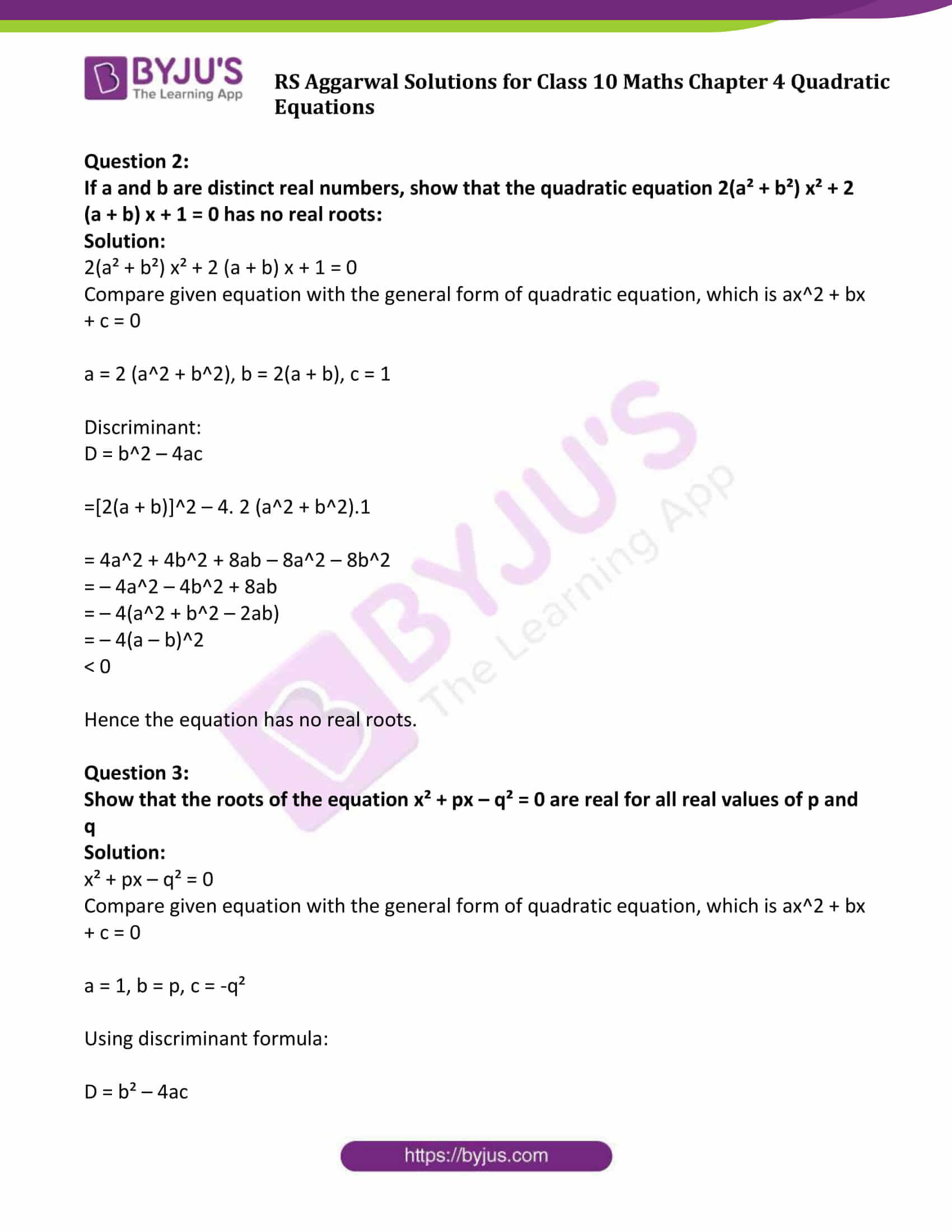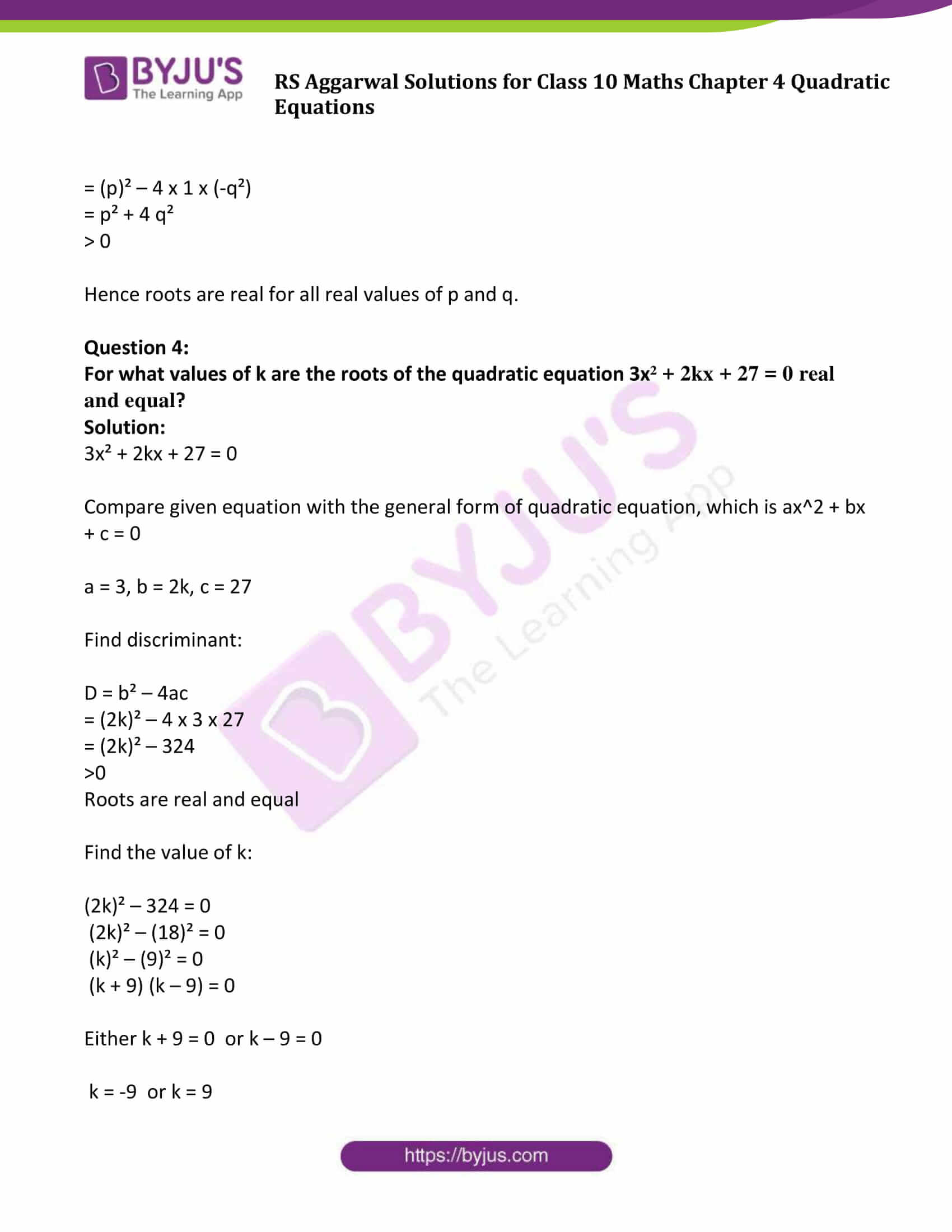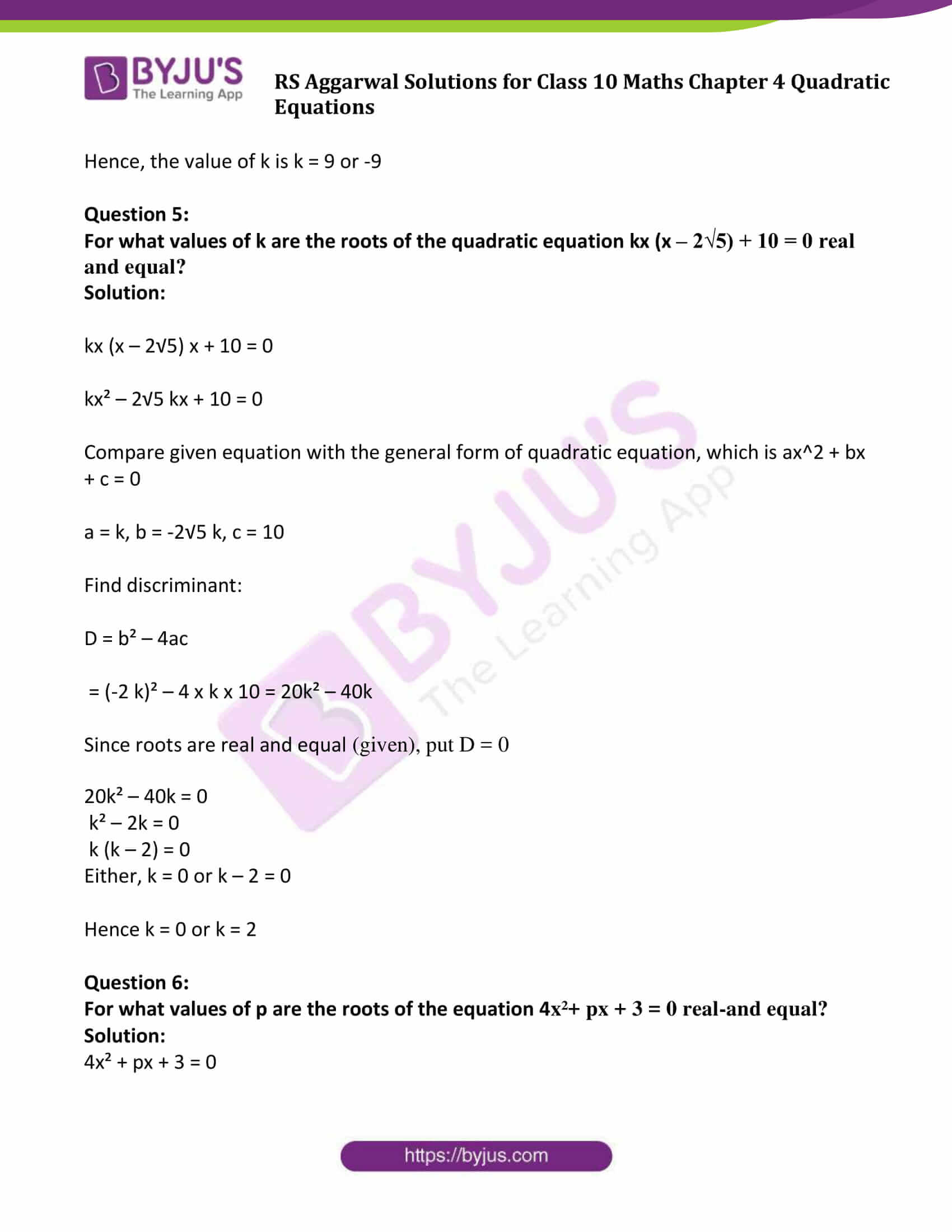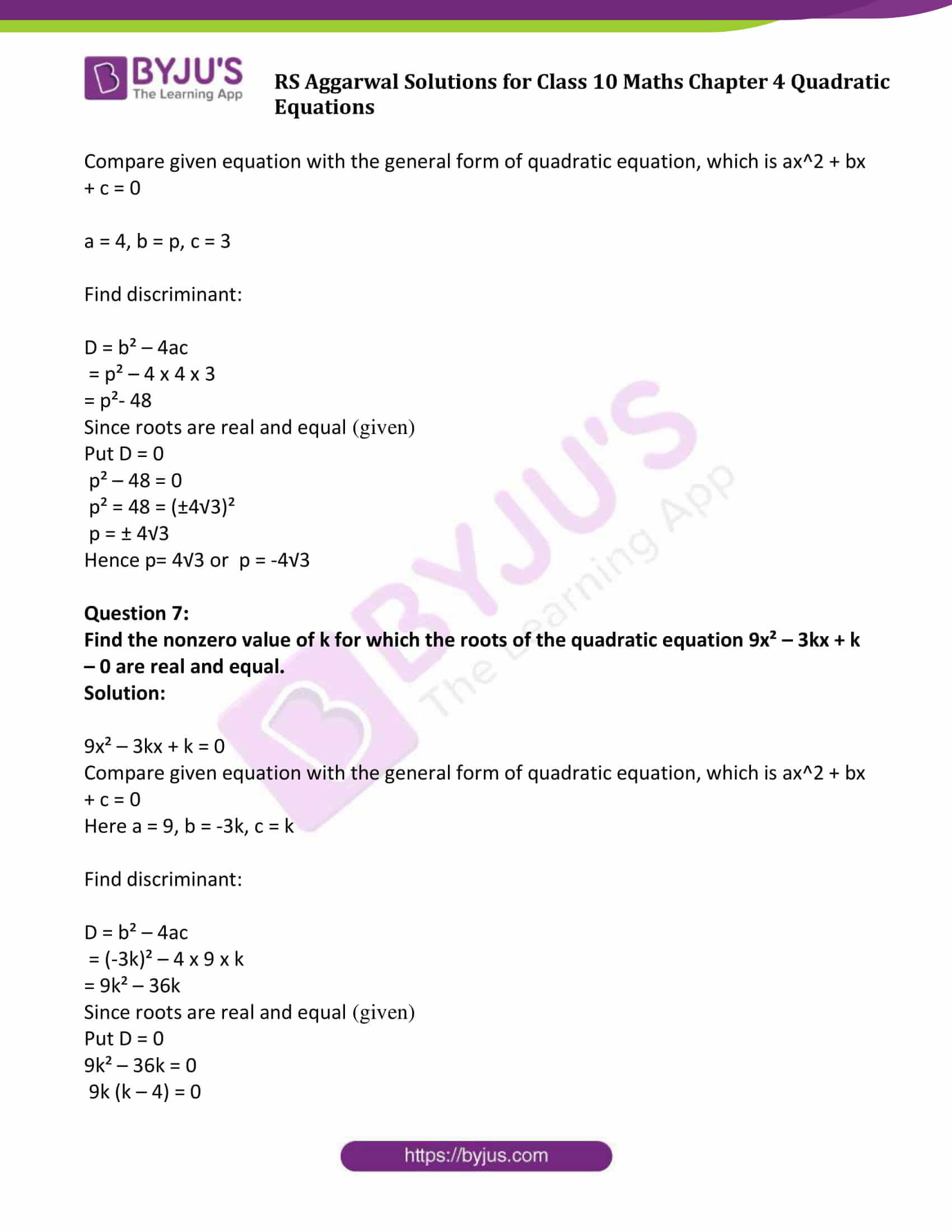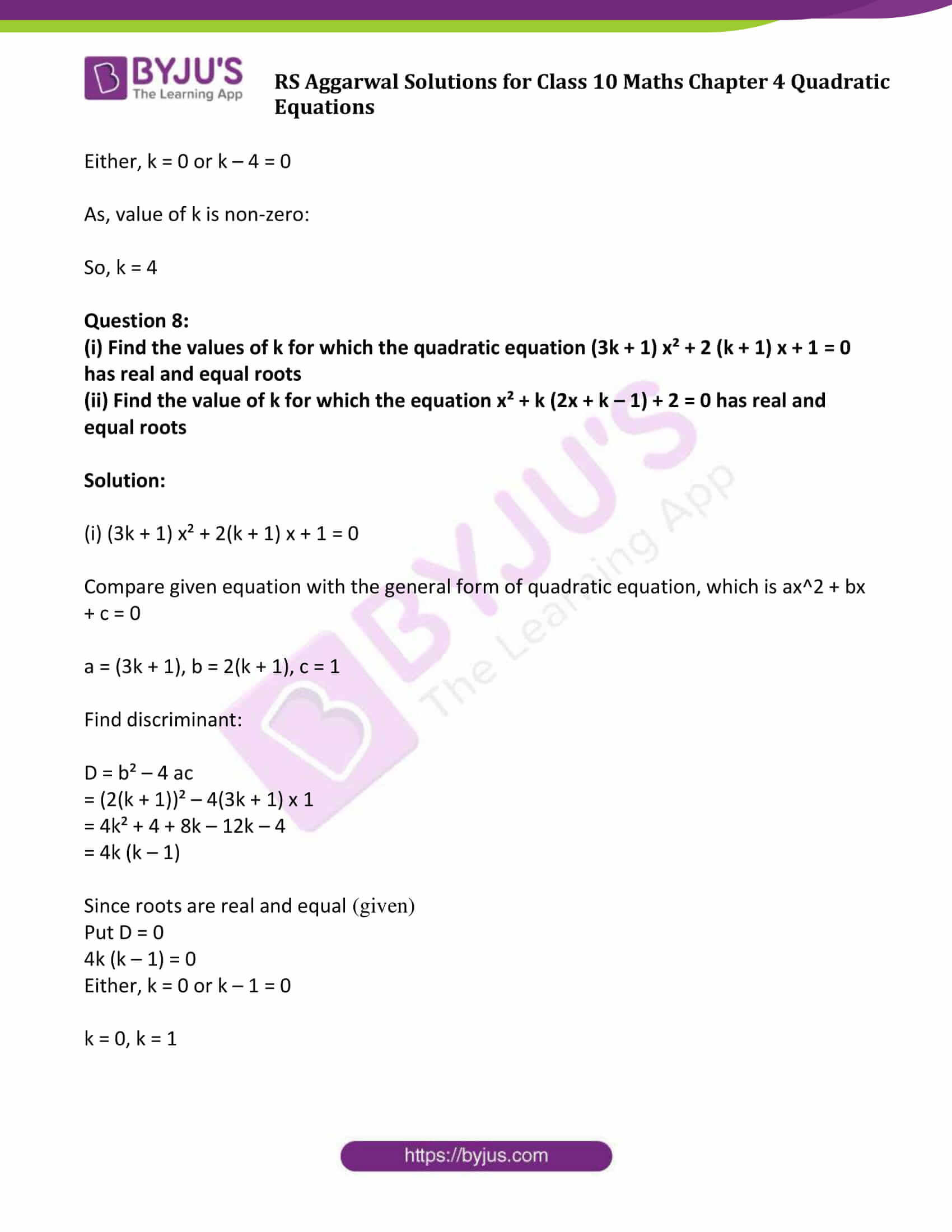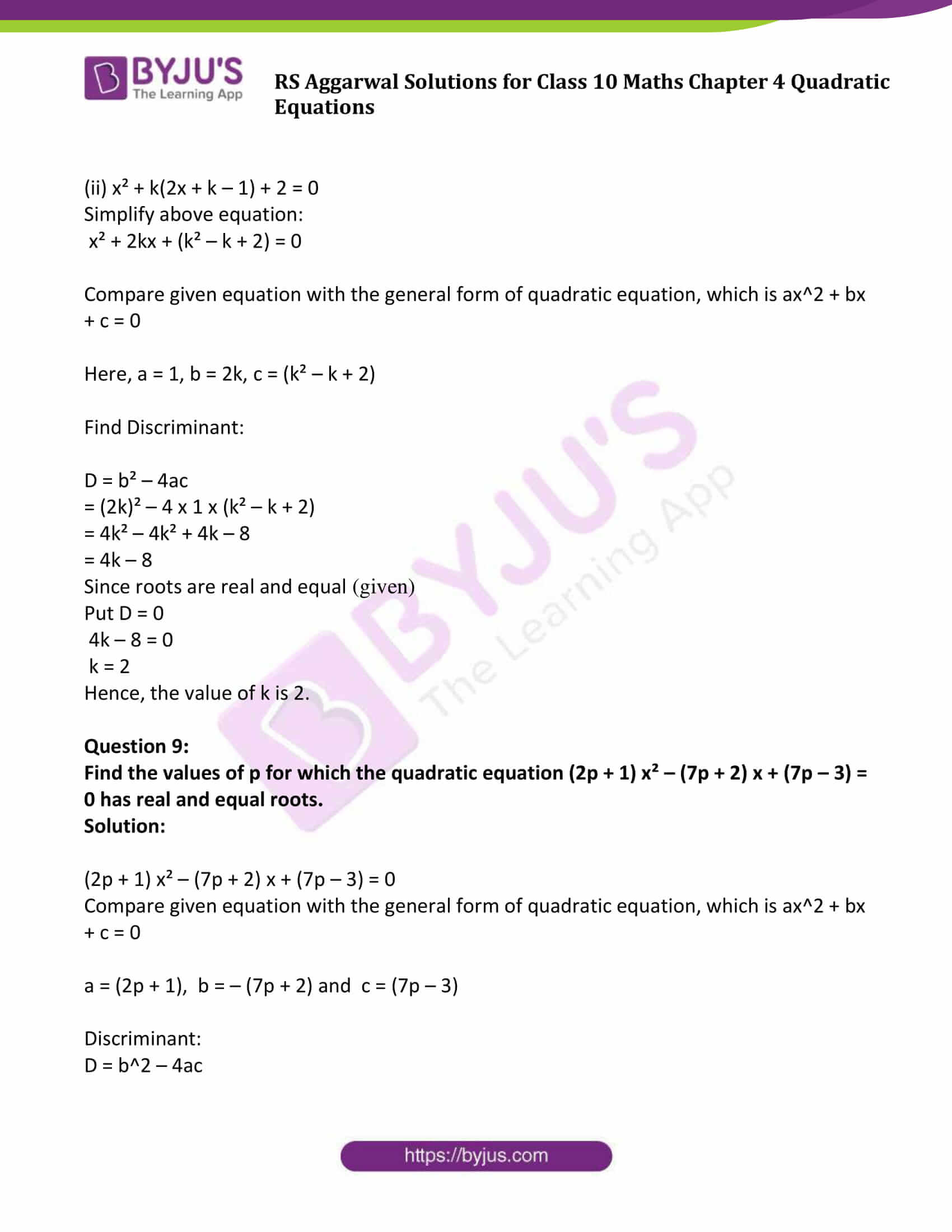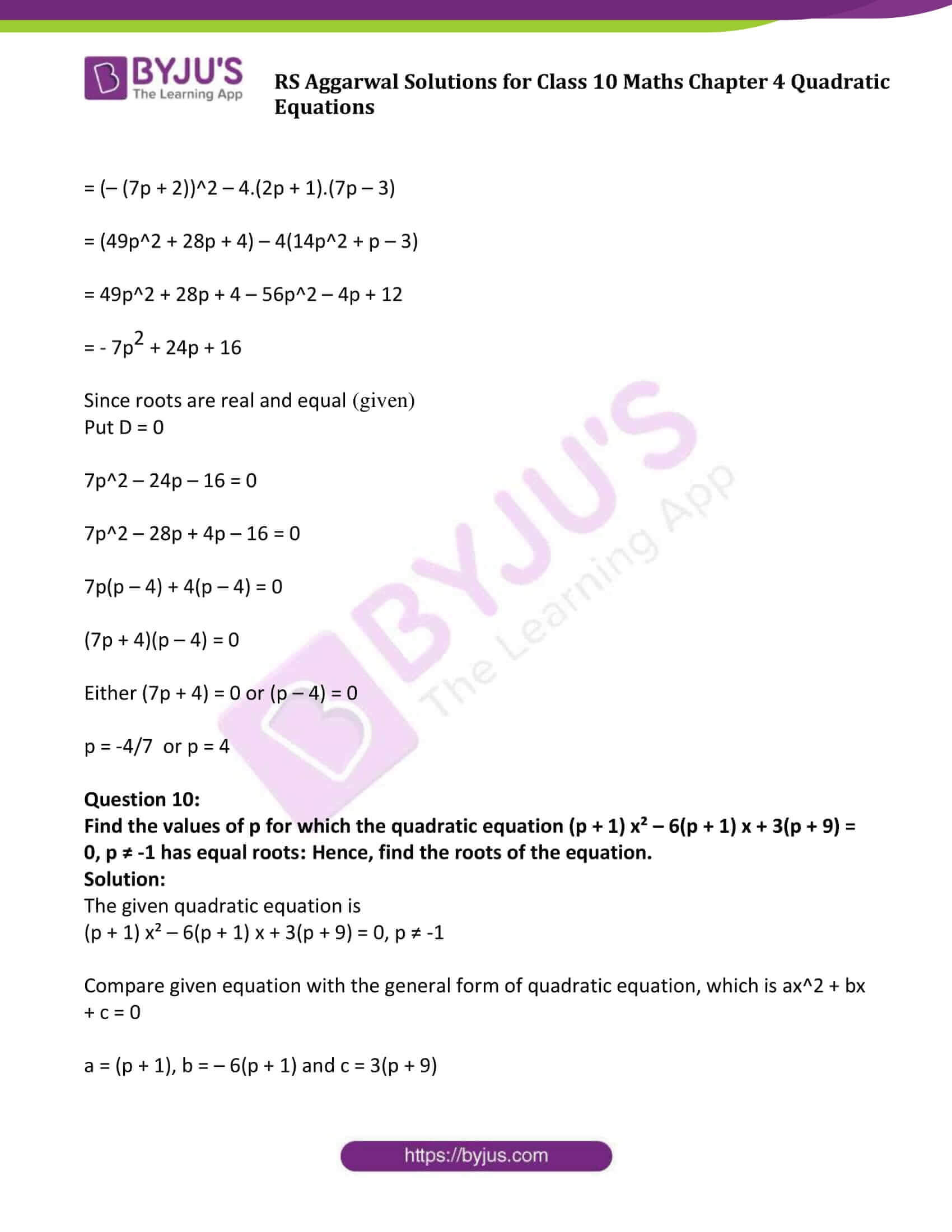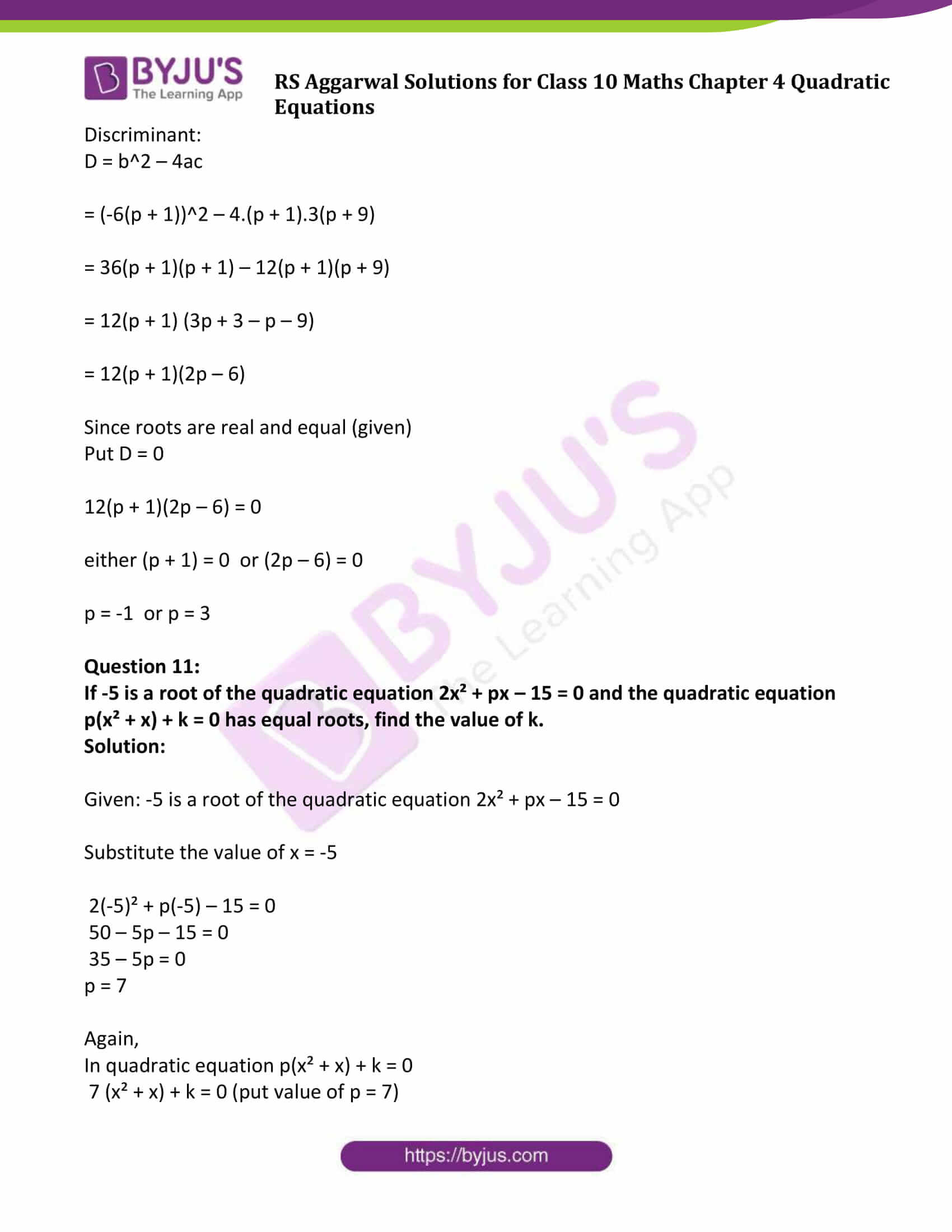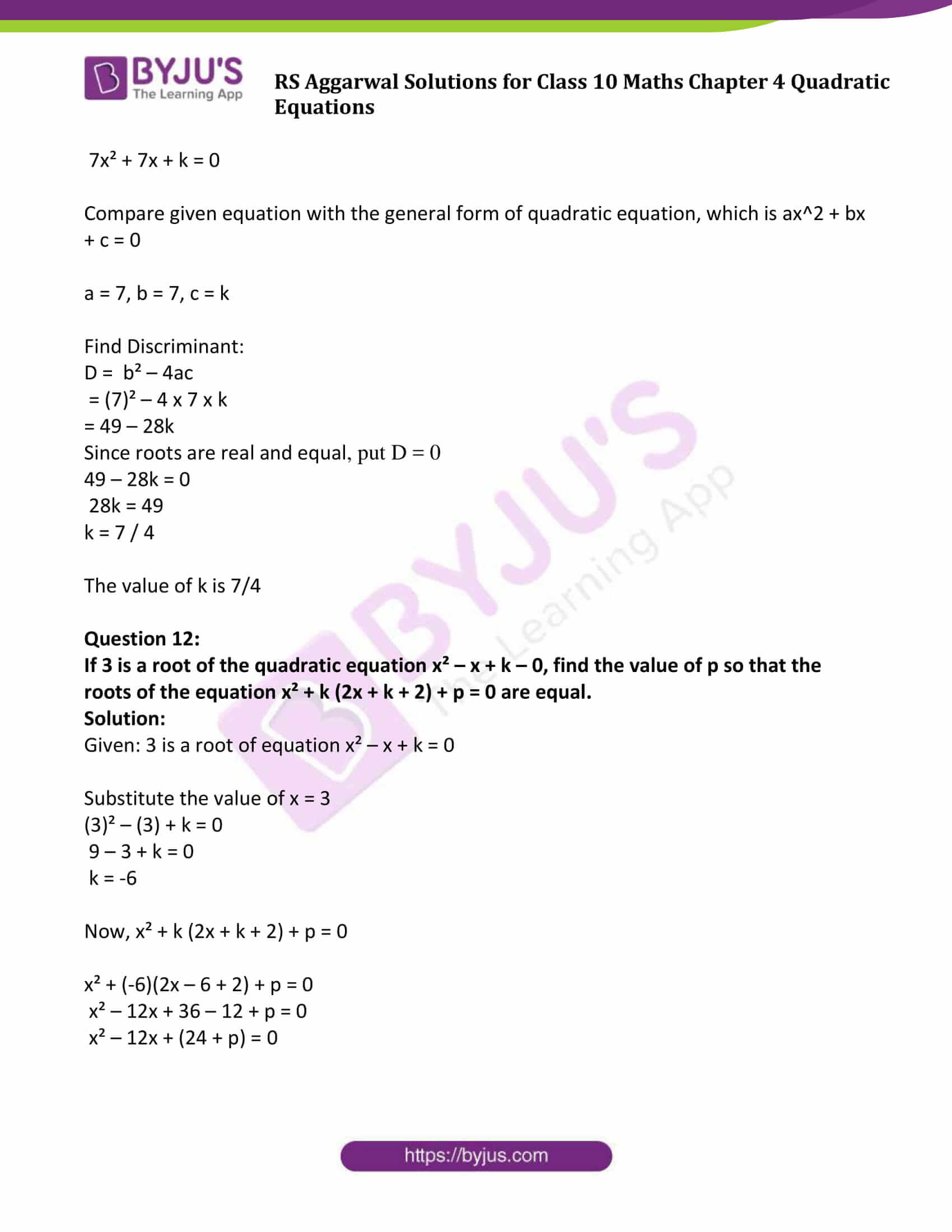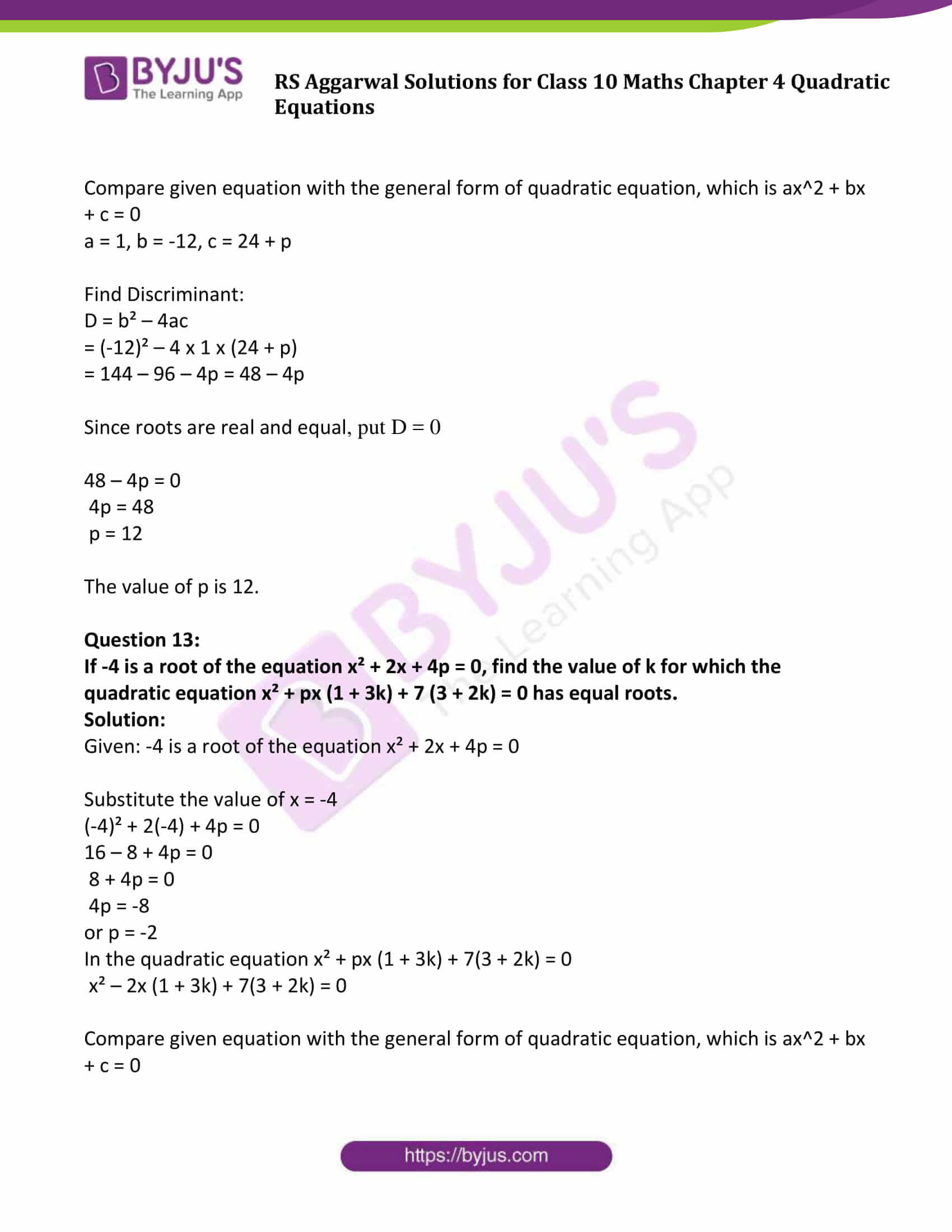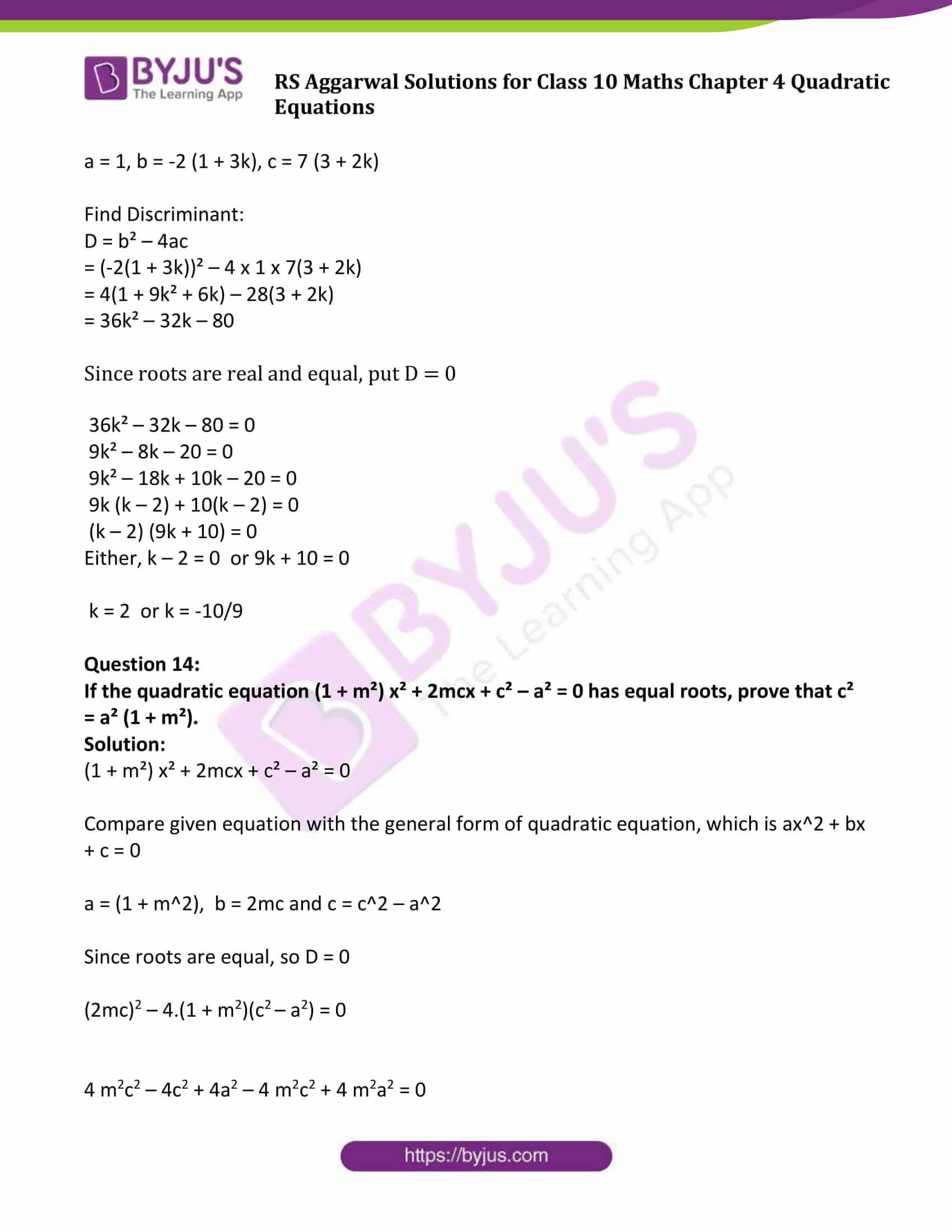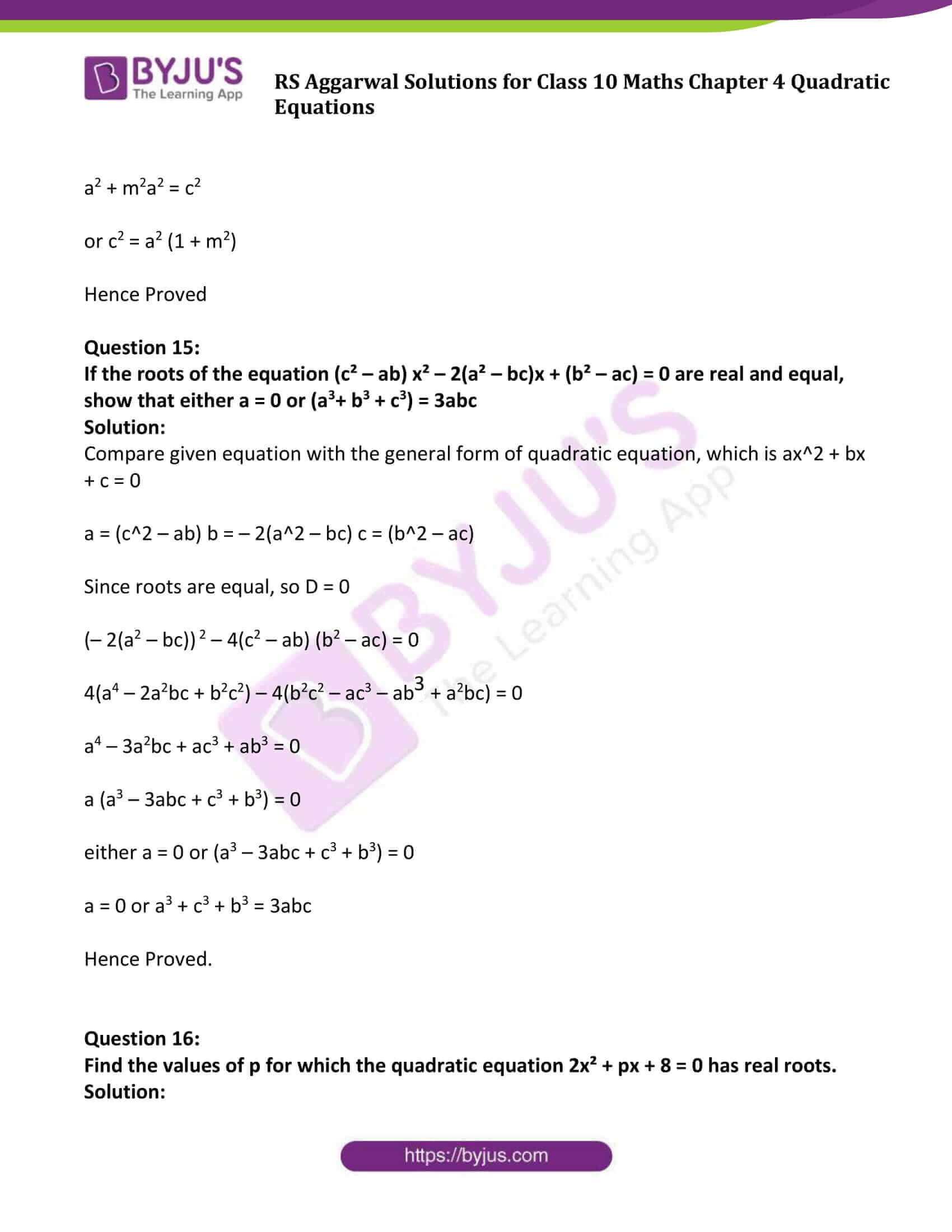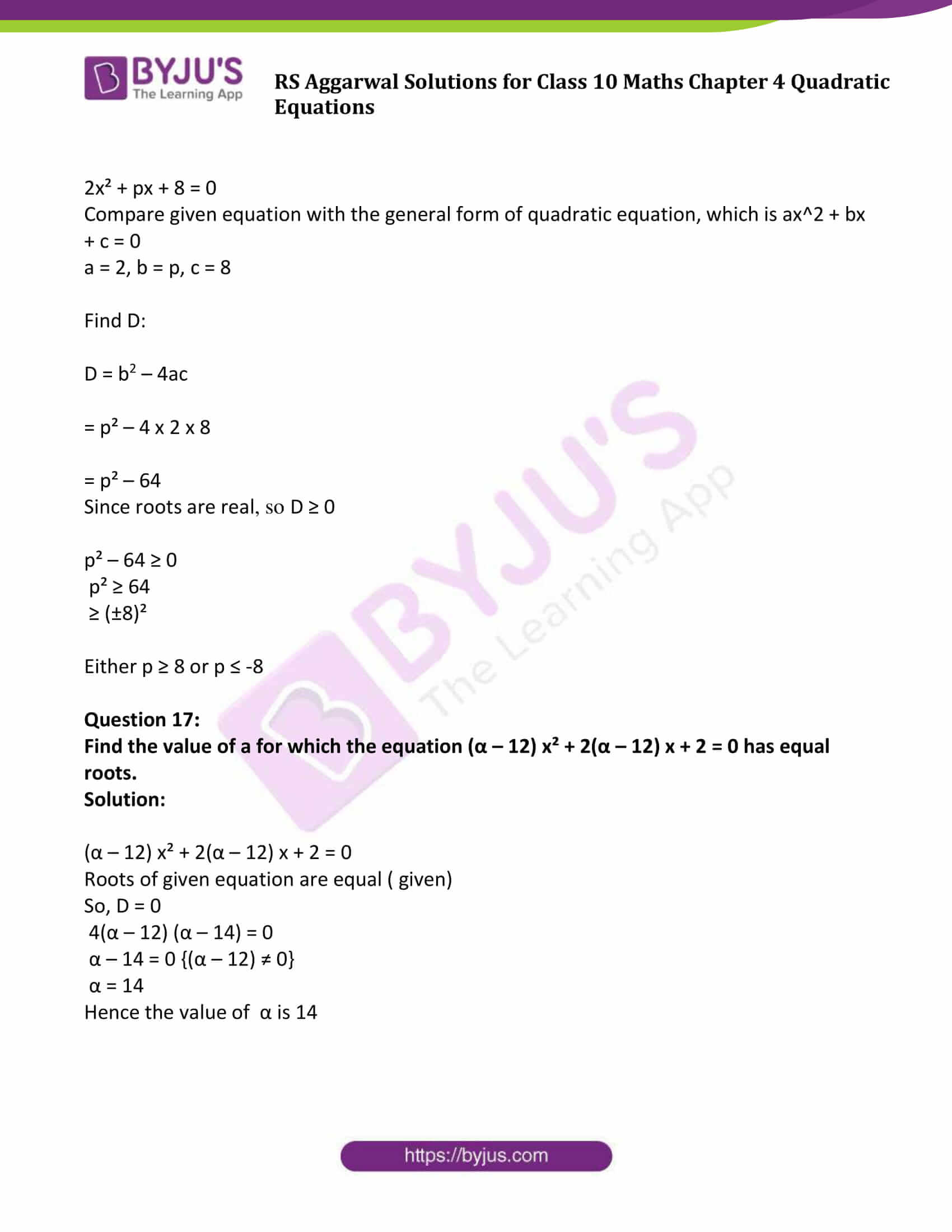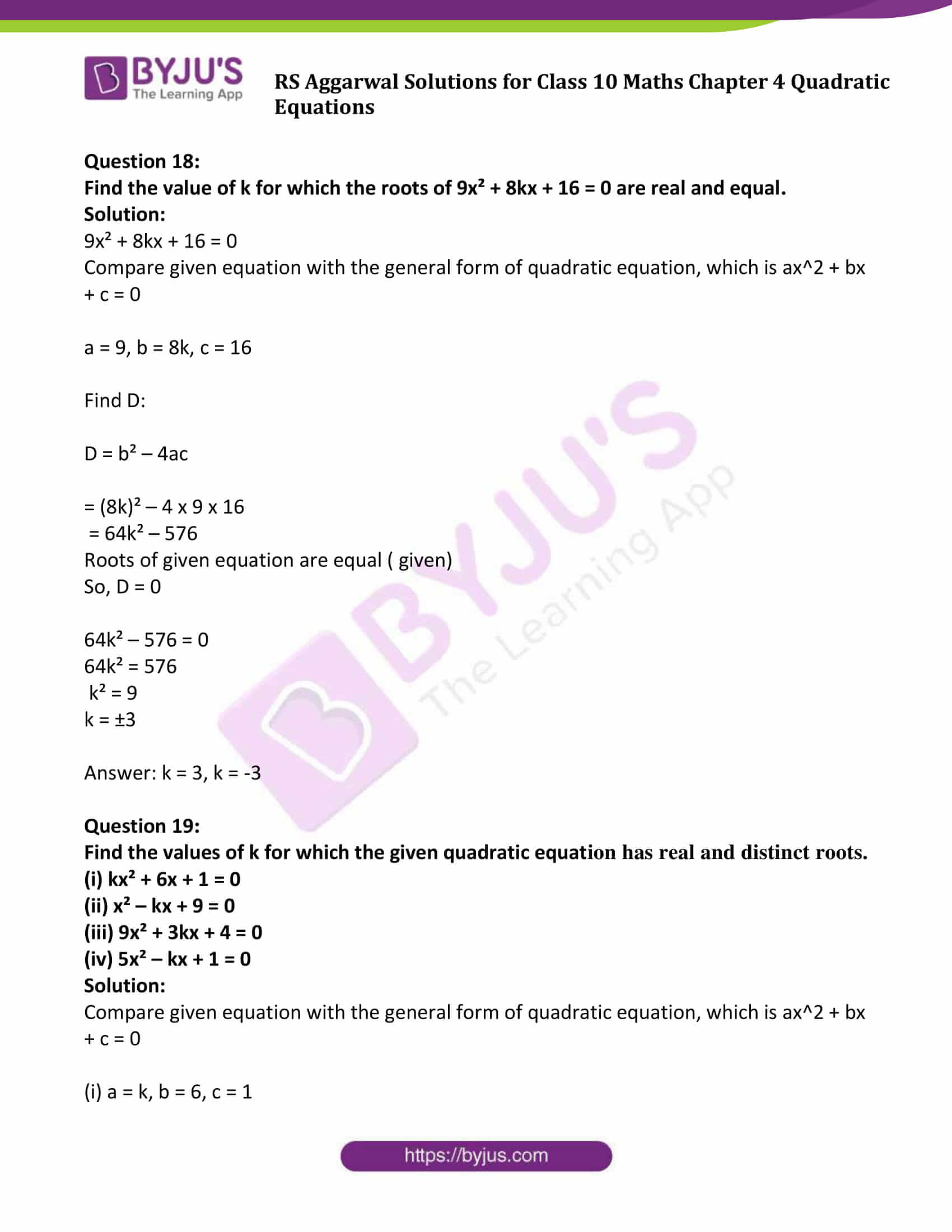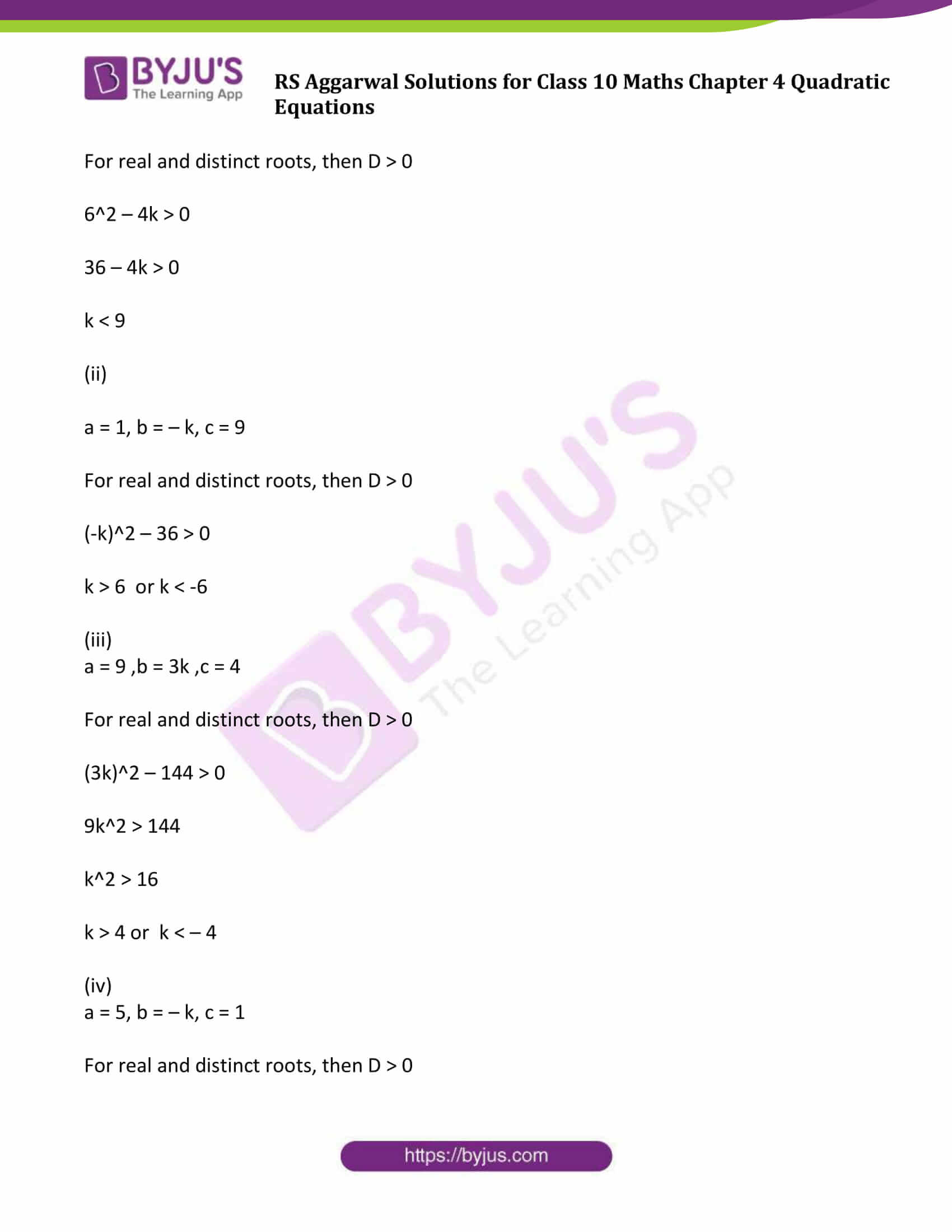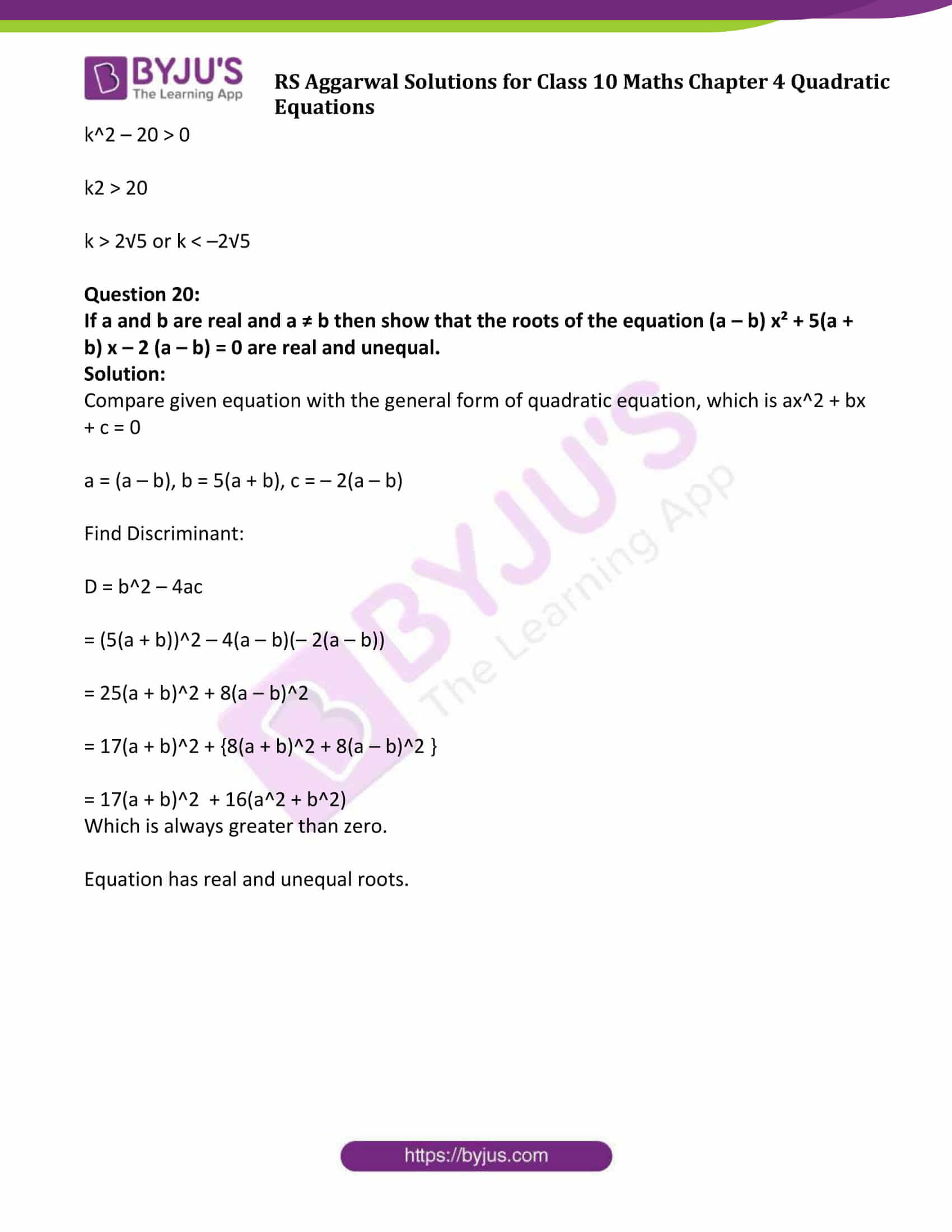### Access Other Exercise solutions of Maths R S Aggarwal Class 10 Chapter 4 – Quadratic Equations

Exercise 4A Solutions : 30 Questions (Short Answers)

Exercise 4B Solutions : 16 Questions (Short Answers)

Exercise 4C Solutions : 15 Questions (Short Answers)

## Exercise 4D Page No: 199

Question 1:

Find the nature of the roots of the following quadratic equations:

(i) 2x² – 8x + 5 = 0

(ii) 3x² – 2√6 x + 2 = 0

(iii) 5x² – 4x + 1 = 0

(iv) 5x (x – 2) + 6 = 0

(v) 12x² – 4√15 x + 5 = 0

(vi) x² – x + 2 = 0

Solution:

(i)2x² – 8x + 5 = 0

Compare given equation with the general form of quadratic equation, which is ax^2 + bx + c = 0

a = 2, b = – 8, c = 5

Using Discriminant Formula:

D = b^2 – 4ac

= (– 8)^2 – 4.2.5

= 64 – 40

= 24 > 0

Hence the roots of equation are real and unequal.

(ii) 3x² – 2√6 x + 2 = 0

Compare given equation with the general form of quadratic equation, which is ax^2 + bx + c = 0

a = 3, b = – 2√6, c = 2

Using Discriminant Formula:

D = b^2 – 4ac

= (– 2√6)^2 – 4.3.2

=24 – 24

= 0

Roots of equation are real and equal.

(iii) 5x² – 4x + 1 = 0

Compare given equation with the general form of quadratic equation, which is ax^2 + bx + c = 0

a = 5, b = – 4, c = 1

Discriminant:

D = b^2 – 4ac

= (– 4)^2 – 4.5.1

= 16 – 20

= – 4 < 0

Equation has no real roots.

(iv) 5x (x – 2) + 6 = 0

5x^2 – 10x + 6 = 0

Compare given equation with the general form of quadratic equation, which is ax^2 + bx + c = 0

a = 5, b = – 10, c = 6

Discriminant:

D = b^2 – 4ac

= (– 10)2 – 4.5.6

= 100 – 120

= – 20 < 0

Equation has no real roots.

(v) 12x² – 4√15 x + 5 = 0

12x² – 4√15 x + 5 = 0

Compare given equation with the general form of quadratic equation, which is ax^2 + bx + c = 0

a = 12, b = – 4√15, c = 5

Discriminant:

D = b^2 – 4ac

= (– 4√15)^2 – 4.12.5

= 240 – 240

= 0

Equation has real and equal roots.

(vi) x² – x + 2 = 0

Compare given equation with the general form of quadratic equation, which is ax^2 + bx + c = 0

a = 1, b = – 1, c = 2

Discriminant:

D = b^2 – 4ac

= (– 1)^2 – 4.1.2

= 1 – 8

= – 7 < 0

Equation has no real roots.

Question 2:

If a and b are distinct real numbers, show that the quadratic equation 2(a² + b²) x² + 2 (a + b) x + 1 = 0 has no real roots:

Solution:

2(a² + b²) x² + 2 (a + b) x + 1 = 0

Compare given equation with the general form of quadratic equation, which is ax^2 + bx + c = 0

a = 2 (a^2 + b^2), b = 2(a + b), c = 1

Discriminant:

D = b^2 – 4ac

=[2(a + b)]^2 – 4. 2 (a^2 + b^2).1

= 4a^2 + 4b^2 + 8ab – 8a^2 – 8b^2

= – 4a^2 – 4b^2 + 8ab

= – 4(a^2 + b^2 – 2ab)

= – 4(a – b)^2

< 0

Hence the equation has no real roots.

Question 3:

Show that the roots of the equation x² + px – q² = 0 are real for all real values of p and q

Solution:

x² + px – q² = 0

Compare given equation with the general form of quadratic equation, which is ax^2 + bx + c = 0

a = 1, b = p, c = -q²

Using discriminant formula:

D = b² – 4ac

= (p)² – 4 x 1 x (-q²)

= p² + 4 q² > 0

Hence roots are real for all real values of p and q.

Question 4:

For what values of k are the roots of the quadratic equation 3x² + 2kx + 27 = 0 real and equal?

Solution:

3x² + 2kx + 27 = 0

Compare given equation with the general form of quadratic equation, which is ax^2 + bx + c = 0

a = 3, b = 2k, c = 27

Find discriminant:

D = b² – 4ac

= (2k)² – 4 x 3 x 27

= (2k)² – 324 >0

Roots are real and equal

Find the value of k:

(2k)² – 324 = 0

(2k)² – (18)² = 0

(k)² – (9)² = 0

(k + 9) (k – 9) = 0

Either k + 9 = 0 or k – 9 = 0

k = -9 or k = 9

Hence, the value of k is k = 9 or -9

Question 5:

For what values of k are the roots of the quadratic equation kx (x – 2√5) + 10 = 0 real and equal?

Solution:

kx (x – 2√5) x + 10 = 0

kx² – 2√5 kx + 10 = 0

Compare given equation with the general form of quadratic equation, which is ax^2 + bx + c = 0

a = k, b = -2√5 k, c = 10

Find discriminant:

D = b² – 4ac

= (-2 k)² – 4 x k x 10 = 20k² – 40k

Since roots are real and equal (given), put D = 0

20k² – 40k = 0

k² – 2k = 0

k (k – 2) = 0

Either, k = 0 or k – 2 = 0

Hence k = 0 or k = 2

Question 6:

For what values of p are the roots of the equation 4x²+ px + 3 = 0 real-and equal?

Solution:

4x² + px + 3 = 0

Compare given equation with the general form of quadratic equation, which is ax^2 + bx + c = 0

a = 4, b = p, c = 3

Find discriminant:

D = b² – 4ac

= p² – 4 x 4 x 3

= p²- 48

Since roots are real and equal (given)

Put D = 0

p² – 48 = 0

p² = 48 = (±4√3)²

p = ± 4√3

Hence p= 4√3 or p = -4√3

Question 7:

Find the nonzero value of k for which the roots of the quadratic equation 9x² – 3kx + k – 0 are real and equal.

Solution:

9x² – 3kx + k = 0

Compare given equation with the general form of quadratic equation, which is ax^2 + bx + c = 0

Here a = 9, b = -3k, c = k

Find discriminant:

D = b² – 4ac

= (-3k)² – 4 x 9 x k

= 9k² – 36k

Since roots are real and equal (given)

Put D = 0

9k² – 36k = 0

9k (k – 4) = 0

Either, k = 0 or k – 4 = 0

As, value of k is non-zero:

So, k = 4

Question 8:

(i) Find the values of k for which the quadratic equation (3k + 1) x² + 2 (k + 1) x + 1 = 0 has real and equal roots

(ii) Find the value of k for which the equation x² + k (2x + k – 1) + 2 = 0 has real and equal roots

Solution:

(i) (3k + 1) x² + 2(k + 1) x + 1 = 0

Compare given equation with the general form of quadratic equation, which is ax^2 + bx + c = 0

a = (3k + 1), b = 2(k + 1), c = 1

Find discriminant:

D = b² – 4 ac

= (2(k + 1))² – 4(3k + 1) x 1

= 4k² + 4 + 8k – 12k – 4

= 4k (k – 1)

Since roots are real and equal (given)

Put D = 0

4k (k – 1) = 0

Either, k = 0 or k – 1 = 0

k = 0, k = 1

(ii) x² + k(2x + k – 1) + 2 = 0

Simplify above equation:

x² + 2kx + (k² – k + 2) = 0

Compare given equation with the general form of quadratic equation, which is ax^2 + bx + c = 0

Here, a = 1, b = 2k, c = (k² – k + 2)

Find Discriminant:

D = b² – 4ac

= (2k)² – 4 x 1 x (k² – k + 2)

= 4k² – 4k² + 4k – 8

= 4k – 8

Since roots are real and equal (given)

Put D = 0

4k – 8 = 0

k = 2

Hence, the value of k is 2.

Question 9:

Find the values of p for which the quadratic equation (2p + 1) x² – (7p + 2) x + (7p – 3) = 0 has real and equal roots.

Solution:

(2p + 1) x² – (7p + 2) x + (7p – 3) = 0

Compare given equation with the general form of quadratic equation, which is ax^2 + bx + c = 0

a = (2p + 1), b = – (7p + 2) and c = (7p – 3)

Discriminant:

D = b^2 – 4ac

= (– (7p + 2))^2 – 4.(2p + 1).(7p – 3)

= (49p^2 + 28p + 4) – 4(14p^2 + p – 3)

= 49p^2 + 28p + 4 – 56p^2 – 4p + 12

= – 7p2 + 24p + 16

Since roots are real and equal (given)

Put D = 0

7p^2 – 24p – 16 = 0

7p^2 – 28p + 4p – 16 = 0

7p(p – 4) + 4(p – 4) = 0

(7p + 4)(p – 4) = 0

Either (7p + 4) = 0 or (p – 4) = 0

p = -4/7 or p = 4

Question 10:

Find the values of p for which the quadratic equation (p + 1) x² – 6(p + 1) x + 3(p + 9) = 0, p ≠ -1 has equal roots: Hence, find the roots of the equation.

Solution:

(p + 1) x² – 6(p + 1) x + 3(p + 9) = 0, p ≠ -1

Compare given equation with the general form of quadratic equation, which is ax^2 + bx + c = 0

a = (p + 1), b = – 6(p + 1) and c = 3(p + 9)

Discriminant:

D = b^2 – 4ac

= (-6(p + 1))^2 – 4.(p + 1).3(p + 9)

= 36(p + 1)(p + 1) – 12(p + 1)(p + 9)

= 12(p + 1) (3p + 3 – p – 9)

= 12(p + 1)(2p – 6)

Since roots are real and equal (given)

Put D = 0

12(p + 1)(2p – 6) = 0

either (p + 1) = 0 or (2p – 6) = 0

p = -1 or p = 3

Question 11:

If -5 is a root of the quadratic equation 2x² + px – 15 = 0 and the quadratic equation p(x² + x) + k = 0 has equal roots, find the value of k.

Solution:

Given: -5 is a root of the quadratic equation 2x² + px – 15 = 0

Substitute the value of x = -5

2(-5)² + p(-5) – 15 = 0

50 – 5p – 15 = 0

35 – 5p = 0

p = 7

Again,

In quadratic equation p(x² + x) + k = 0

7 (x² + x) + k = 0 (put value of p = 7)

7x² + 7x + k = 0

Compare given equation with the general form of quadratic equation, which is ax^2 + bx + c = 0

a = 7, b = 7, c = k

Find Discriminant:

D = b² – 4ac

= (7)² – 4 x 7 x k

= 49 – 28k

Since roots are real and equal, put D = 0

49 – 28k = 0

28k = 49

k = 7 / 4

The value of k is 7/4

Question 12:

If 3 is a root of the quadratic equation x² – x + k – 0, find the value of p so that the roots of the equation x² + k (2x + k + 2) + p = 0 are equal.

Solution:

Given: 3 is a root of equation x² – x + k = 0

Substitute the value of x = 3

(3)² – (3) + k = 0

9 – 3 + k = 0

k = -6

Now, x² + k (2x + k + 2) + p = 0

x² + (-6)(2x – 6 + 2) + p = 0

x² – 12x + 36 – 12 + p = 0

x² – 12x + (24 + p) = 0

Compare given equation with the general form of quadratic equation, which is ax^2 + bx + c = 0

a = 1, b = -12, c = 24 + p

Find Discriminant:

D = b² – 4ac

= (-12)² – 4 x 1 x (24 + p)

= 144 – 96 – 4p = 48 – 4p

Since roots are real and equal, put D = 0

48 – 4p = 0

4p = 48

p = 12

The value of p is 12.

Question 13:

If -4 is a root of the equation x² + 2x + 4p = 0, find the value of k for which the quadratic equation x² + px (1 + 3k) + 7 (3 + 2k) = 0 has equal roots.

Solution:

Given: -4 is a root of the equation x² + 2x + 4p = 0

Substitute the value of x = -4

(-4)² + 2(-4) + 4p = 0

16 – 8 + 4p = 0

8 + 4p = 0

4p = -8

or p = -2

In the quadratic equation x² + px (1 + 3k) + 7(3 + 2k) = 0

x² – 2x (1 + 3k) + 7(3 + 2k) = 0

Compare given equation with the general form of quadratic equation, which is ax^2 + bx + c = 0

a = 1, b = -2 (1 + 3k), c = 7 (3 + 2k)

Find Discriminant:

D = b² – 4ac

= (-2(1 + 3k))² – 4 x 1 x 7(3 + 2k)

= 4(1 + 9k² + 6k) – 28(3 + 2k)

= 36k² – 32k – 80

Since roots are real and equal, put D = 0

36k² – 32k – 80 = 0

9k² – 8k – 20 = 0

9k² – 18k + 10k – 20 = 0

9k (k – 2) + 10(k – 2) = 0

(k – 2) (9k + 10) = 0

Either, k – 2 = 0 or 9k + 10 = 0

k = 2 or k = -10/9

Question 14:

If the quadratic equation (1 + m²) x² + 2mcx + c² – a² = 0 has equal roots, prove that c² = a² (1 + m²).

Solution:

(1 + m²) x² + 2mcx + c² – a² = 0

Compare given equation with the general form of quadratic equation, which is ax^2 + bx + c = 0

a = (1 + m^2), b = 2mc and c = c^2 – a^2

Since roots are equal, so D = 0

(2mc)2 – 4.(1 + m2)(c2 – a2) = 0

4 m2c2 – 4c2 + 4a2 – 4 m2c2 + 4 m2a2 = 0

a2 + m2a2 = c2

or c2 = a2 (1 + m2)

Hence Proved

Question 15:

If the roots of the equation (c² – ab) x² – 2(a² – bc)x + (b² – ac) = 0 are real and equal, show that either a = 0 or (a3+ b3 + c3) = 3abc

Solution:

Compare given equation with the general form of quadratic equation, which is ax^2 + bx + c = 0

a = (c^2 – ab) b = – 2(a^2 – bc) c = (b^2 – ac)

Since roots are equal, so D = 0

(– 2(a2 – bc)) 2 – 4(c2 – ab) (b2 – ac) = 0

4(a4 – 2a2bc + b2c2) – 4(b2c2 – ac3 – ab3 + a2bc) = 0

a4 – 3a2bc + ac3 + ab3 = 0

a (a3 – 3abc + c3 + b3) = 0

either a = 0 or (a3 – 3abc + c3 + b3) = 0

a = 0 or a3 + c3 + b3 = 3abc

Hence Proved.

Question 16:

Find the values of p for which the quadratic equation 2x² + px + 8 = 0 has real roots.

Solution:

2x² + px + 8 = 0

Compare given equation with the general form of quadratic equation, which is ax^2 + bx + c = 0

a = 2, b = p, c = 8

Find D:

D = b2 – 4ac

= p² – 4 x 2 x 8

= p² – 64

Since roots are real, so D ≥ 0

p² – 64 ≥ 0

p² ≥ 64

≥ (±8)²

Either p ≥ 8 or p ≤ -8

Question 17:

Find the value of a for which the equation (α – 12) x² + 2(α – 12) x + 2 = 0 has equal roots.

Solution:

(α – 12) x² + 2(α – 12) x + 2 = 0

Roots of given equation are equal ( given)

So, D = 0

4(α – 12) (α – 14) = 0

α – 14 = 0 {(α – 12) ≠ 0}

α = 14

Hence the value of α is 14

Question 18:

Find the value of k for which the roots of 9x² + 8kx + 16 = 0 are real and equal.

Solution:

9x² + 8kx + 16 = 0

Compare given equation with the general form of quadratic equation, which is ax^2 + bx + c = 0

a = 9, b = 8k, c = 16

Find D:

D = b² – 4ac

= (8k)² – 4 x 9 x 16

= 64k² – 576

Roots of given equation are equal ( given)

So, D = 0

64k² – 576 = 0

64k² = 576

k² = 9

k = ±3

Answer: k = 3, k = -3

Question 19:

Find the values of k for which the given quadratic equation has real and distinct roots.

(i) kx² + 6x + 1 = 0

(ii) x² – kx + 9 = 0

(iii) 9x² + 3kx + 4 = 0

(iv) 5x² – kx + 1 = 0

Solution:

Compare given equation with the general form of quadratic equation, which is ax^2 + bx + c = 0

(i) a = k, b = 6, c = 1

For real and distinct roots, then D > 0

6^2 – 4k > 0

36 – 4k > 0

k < 9

(ii)

a = 1, b = – k, c = 9

For real and distinct roots, then D > 0

(-k)^2 – 36 > 0

k > 6 or k < -6

(iii)

a = 9 ,b = 3k ,c = 4

For real and distinct roots, then D > 0

(3k)^2 – 144 > 0

9k^2 > 144

k^2 > 16

k > 4 or k < – 4

(iv)

a = 5, b = – k, c = 1

For real and distinct roots, then D > 0

k^2 – 20 > 0

k2 > 20

k > 2√5 or k < –2√5

Question 20:

If a and b are real and a ≠ b then show that the roots of the equation (a – b) x² + 5(a + b) x – 2 (a – b) = 0 are real and unequal.

Solution:

Compare given equation with the general form of quadratic equation, which is ax^2 + bx + c = 0

a = (a – b), b = 5(a + b), c = – 2(a – b)

Find Discriminant:

D = b^2 – 4ac

= (5(a + b))^2 – 4(a – b)(– 2(a – b))

= 25(a + b)^2 + 8(a – b)^2

= 17(a + b)^2 + {8(a + b)^2 + 8(a – b)^2 }

= 17(a + b)^2 + 16(a^2 + b^2)

Which is always greater than zero.

Equation has real and unequal roots.

## R S Aggarwal Solutions for Class 10 Maths Chapter 4 Quadratic Equations Exercise 4D

Class 10 Maths Chapter 4 Quadratic Equations Exercise 4D is based on the nature of roots of a quadratic equation.

Discriminant is represented as D. Formula to find the D is D = b^2-4ac.

• Case 1: When D>0

Roots are real and distinct.

• Case 2: When D = 0

Roots are real and equal.

• Case 3: D < 0

Roots are imaginary. Also we can say that the equation has no real roots.

You can also practice numerous problems on R S Aggarwal Class 10 Maths and master quadratic equations.